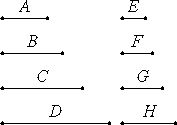# Proposition 1

If there are as many numbers as we please in continued proportion, and the extremes of them are relatively prime, then the numbers are the least of those which have the same ratio with them.

Let there be as many numbers as we please, A, B, C, and D, in continued proportion, and let the extremes of them, A and D, be relatively prime.

I say that A, B, C, and D are the least of those which have the same ratio with them.

If not, let E, F, G, and H be less than A, B, C, and D, and in the same ratio with them.VII.14

Now, since A, B, C, and D are in the same ratio with E, F, G, and H, and the multitude of the numbers A, B, C, and D equals the multitude of the numbers E, F, G, and H, therefore, ex aequali A is to D as E is to H.

But A and D are relatively prime, numbers which are relatively prime are also least, and the least numbers measure those which have the same ratio the same number of times, the greater the greater and the less the less, that is, the antecedent the antecedent and the consequent the consequent. Therefore A measures E, the greater the less, which is impossible.

Therefore E, F, G, and H, which are less than A, B, C, and D, are not in the same ratio with them. Therefore A, B, C, and D are the least of those which have the same ratio with them.

Therefore, if there are as many numbers as we please in continued proportion, and the extremes of them are relatively prime, then the numbers are the least of those which have the same ratio with them.

Q.E.D.

## Guide

#### Continued proportions and geometric progressions

In this proposition we consider only continued proportions with a constant ratio. They are proportions of the form

a1 : a2 = a2 : a3 = a3 : a4 = ... = an-1 : an.

In proposition VIII.4 continued proportions that don’t have a constant ratio will be considered.

An example of continued proportion with constant ratio is

1250 : 750 = 750 : 450 = 450 : 270 = 270 : 162

since each of the ratios is the same as the ratio 5 : 3.

A modern expression for this situation is to say that the numbers a1, a2, a3, ..., an-1an are in a geometric progression or a geometric sequence. The ratio of any consecutive pair in a geometric progression is constant.

Many of the propositions in Books VIII and IX treat geometric progressions. The sum of a geometric progression is found in proposition IX.35.